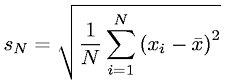Equations > Statistics > Moments > Sample Variance (Biased)

### Sample Variance (Biased)Latex Code:

MathML Code:

 ${s}_{N}=\sqrt{\frac1N\sum _{i=1}^{N}{\left({x}_{i}-\stackrel{‾}{x}\right)}^{2}}$

MathType 5.0: